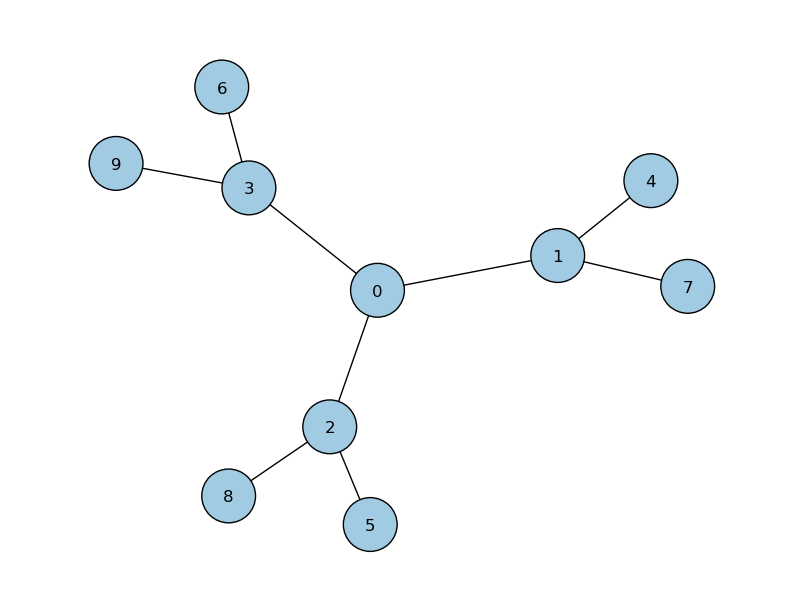# 2P_3cayley.py¶

## Description¶This example propagates a 2 particle continuous-time quantum walk on a 3-cayley tree. Amongst the features used, it illustrates: recieving command line options using PETSc the use of the chebyshev algorithm, with minimum eigenvalue pre-set adding a diagonal defect to various nodes same-node interactions between particles creating node handles to watch the probability at specified nodes creating entanglement handles to watch the entanglement various plotting abilities: probability vs node plots probability vs time plots graph plots entanglement plots outputs the partial trace of the density matrix in binary form

## Source Code¶

  1 2 3 4 5 6 7 8 9 10 11 12 13 14 15 16 17 18 19 20 21 22 23 24 25 26 27 28 29 30 31 32 33 34 35 36 37 38 39 40 41 42 43 44 45 46 47 48 49 50 51 52 53 54 55 56 57 58 59 60 61 62 63 64 65 66 67 68 69 70 71 72 73 74 75 76 77 #!/usr/bin/env python2.7 # initialize PETSc import sys, petsc4py petsc4py.init(sys.argv) from petsc4py import PETSc import numpy as np # import pyCTQW as qw import pyCTQW.MPI as qw # enable command line arguments -t and -N OptDB = PETSc.Options() N = OptDB.getInt('N', 10) t = OptDB.getReal('t', 5) # get the MPI rank rank = PETSc.Comm.Get_rank(PETSc.COMM_WORLD) if rank == 0: print '2P 3-cayley Tree CTQW\n' # initialise a 10 node graph CTQW walk = qw.Graph2P(N) # Create a 2 particle interacting Hamiltonian from a file. # A defect of amplitude 2 and 0.5 has # been added to nodes 3 and 4 respectively. #d = [3,4] #amp = [2.0,1.5] d= amp=[0.] walk.createH('../graphs/cayley/3-cayley.txt','txt',d=d,amp=amp,layout='spring',interaction=0.5,bosonic=True) H=qw.io.matToSparse(walk.H.mat) H=np.real(H.toarray()) np.savetxt('out/bh.txt',H, fmt='%.1f') # create the initial state (1/sqrt(2)) (|0,1>+i|1,1>) init_state = [[0,1,1.0/np.sqrt(2.0)], [1,0,1.0/np.sqrt(2.0)]] walk.createInitState(init_state) # set the eigensolver properties. #Note that Emin has been set exactly. walk.EigSolver.setEigSolver(tol=1.e-2,verbose=False,emin_estimate=0.) # create a handle to watch the probability at nodes 0-4,9: walk.watch([0,1,2,3,4,9]) # create a handle to watch the entanglement walk.watch(None,watchtype='entanglement',verbose=False,esolver='lapack',max_it=100) # Propagate the CTQW using the Chebyshev method # for t=5s in timesteps of dt=0.01 for dt in np.arange(0.01,t+0.01,0.01): walk.propagate(dt,method='chebyshev') # plot the p1 and p2 marginal probabilities # after propagation over all nodes walk.plot('out/2p_3cayley_plot.png') # plot a 3D graph representation with bars # representing the p1 and p2 marginal probabilities walk.plotGraph(output='out/2p_3cayley_graph.png') # plot the p1 and p2 probability over time for watched node1 walk.plotNode('out/2p_3cayley_node1.png',1) # plot the particle 1 probability over all watched nodes walk.plotNodes('out/2p_3cayley_nodes_particle1.png',p=1) # plot the particle 2 probability over all watched nodes walk.plotNodes('out/2p_3cayley_nodes_particle2.png',p=2) # plot the entanglement vs. time walk.plotEntanglement('out/2p_3cayley_ent.png') # export the partial trace walk.exportPartialTrace('out/3-cayley-2p-rhoX.txt','txt',p=1) # destroy the quantum walk walk.destroy()